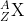×
Get Full Access to Chemistry: A Molecular Approach - 3 Edition - Chapter 2 - Problem 55e
Get Full Access to Chemistry: A Molecular Approach - 3 Edition - Chapter 2 - Problem 55e

×

# The amount of carbon-14 in ancient artifacts and fossilsISBN: 9780321809247 1

## Solution for problem 55E Chapter 2

Chemistry: A Molecular Approach | 3rd Edition

• Textbook Solutions
• 2901 Step-by-step solutions solved by professors and subject experts
• Get 24/7 help from StudySoup virtual teaching assistantsChemistry: A Molecular Approach | 3rd Edition

4 5 1 356 Reviews
26
2
Problem 55E

The amount of carbon-14 in ancient artifacts and fossils is often used to establish their age. Determine the number of protons and the number of neutrons in a carbon-14 isotope and write its symbol in the form.

Step-by-Step Solution:
Step 1 of 3

Solution : Let’s consider the 1st set of data : The given symbol is Co. Cobalt is the chemical name of symbol Co. It has an atomic number of 27. Atomic number indicates the number of protons and electrons. So there are 27 rotons and electrons. But there is an charge of 3+ which indicates there is loss of 3 electrons, Hence number of electrons here is 4. Mass number is given as 59. To find the number of neutrons, we subtract the number of protons from the mass number as mass number is the sum of number of protons and neutrons. Therefore, Number of neutrons = 59- 27 = 3 2. Let’s consider the 2nd set of data : Number of protons is given as 34. It indicates the element’s atomic number is 34. Element with atomic number 34 is Selenium(Se). Number of electrons is given as 36. This indicates there is gain of 2 electrons as in neutral state number of protons and electrons are equal and hence the element has a charge of 2-. Mass number is the sum of protons and neutrons. Hence mass number = 34+46 = 80. Let’s consider the 3rd set of data : Number of protons is given as 76. It indicates the element’s atomic number is 76. Element with atomic number 76 is Osmium(Os). Net charge of the element is given as 2+, which indicates there is loss of 2 electrons. Hence, number of electrons is 74. Mass number is the sum of protons and neutrons. Hence mass number = 76+116 = 192. Let’s consider the 4th set of data : Number of protons is given as 80. It indicates the element’s atomic number is 80. Element with atomic number 80 is Mercury(Hg). Number of electrons is given as 78. This indicates there is loss of 2 electrons and hence the net charge of the element is 2+. Mass number is the sum of protons and neutrons. Hence mass number = 80+120 = 200. So finally with all the above derived data, the table is as follows : Symbol 59Co3+ 8Se2+ 19Os2+ 20Hg2+ Protons 27 34 76 80 Neutrons 32 46 116 120 Electrons 24 36 74 78 Net charge 3+ 2- 2+ 2+

Step 2 of 3

Step 3 of 3

##### ISBN: 9780321809247

Chemistry: A Molecular Approach was written by and is associated to the ISBN: 9780321809247. This textbook survival guide was created for the textbook: Chemistry: A Molecular Approach, edition: 3. The answer to “?The amount of carbon-14 in ancient artifacts and fossils is often used to establish their age. Determine the number of protons and the number of neutrons in a carbon-14 isotope and write its symbol in the form .” is broken down into a number of easy to follow steps, and 38 words. This full solution covers the following key subjects: Table, compounds, Molecules, fill, gaps. This expansive textbook survival guide covers 82 chapters, and 9454 solutions. The full step-by-step solution to problem: 55E from chapter: 2 was answered by , our top Chemistry solution expert on 02/22/17, 04:35PM. Since the solution to 55E from 2 chapter was answered, more than 359 students have viewed the full step-by-step answer.

Unlock Textbook Solution